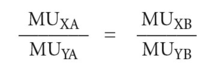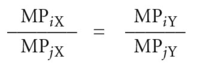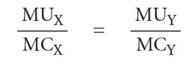# Pareto optimality

(redirected from Pareto efficiency)
Also found in: Encyclopedia, Wikipedia.

## Pareto optimality

the maximization of the economic welfare of the community.

There are three conditions that must hold in order for a Pareto optimum to be attained.

Consider a simplified economy in which there are two goods produced (X and Y) and two consumers (A and B).

1. optimal distribution of goods between consumers requires that:MUYB where MUXA is the MARGINAL UTILITY of good X to consumer A, MUYA is the marginal utility of good Y to consumer A, and so forth. This condition states that the MARGINAL RATE OF SUBSTITUTION between two goods (the ratio of their marginal utilities) must be the same for each consumer. If this were not the case, the consumers could improve their positions by exchanging goods. The consumer who values X highly relative to Y could trade some of his Y to the consumer who values Y highly relative to X. Only when the utility ratios are the same for both consumers is such mutually beneficial trade impossible.

INDIFFERENCE CURVES that show combinations of goods that yield satisfaction to consumers can be used to depict marginal rates of substitution insofar as their slopes reflect these trade-offs.

2. optimal allocation of inputs in productive uses where two inputs i and j are used in the production of goods X and Y. Optimal utilization of inputs requires that the ratio of the MARGINAL PHYSICAL PRODUCTS of i and j employed in the production of X be the same as the ratio of their marginal physical products in the production of Y. That is:If this equality is not satisfied, one input is relatively more efficient in the production of one output (and the other input is thus relatively less efficient in the production of the same output). It will thus benefit the community to divert more of the first input into its more efficient use (and to divert the other input from that use). This will permit the community to expand the production of one output at a constant level of resource use. Only when the marginal product ratios are the same can the community not gain by reallocating its inputs among competing uses. Production ISOQUANTS, which show combinations of inputs that produce a given output, can be used to depict MARGINAL RATES OF TECHNICAL SUBSTITUTION insofar as their slopes reflect these trade-offs.

3. optimal amounts of output (illustrated in Fig. 139, which depicts a transformation curve (PRODUCTION POSSIBILITY BOUNDARY) shows the quantities of goods X and Y that can be produced by utilizing fully the resources of the community). The slope of this transformation curve represents the MARGINAL RATE OF TRANSFORMATION, that is, the ratio of the MARGINAL COST of good X to the marginal cost of good Y. Optimal output for any pair of goods, X and Y, requires that the goods be produced in quantities such that:that is, the ratio of marginal cost to marginal utility must be the same for each good, so that the last £'s worth of good X generates the same utility as the last £'s worth of good Y, etc. This condition will be satisfied if the slope of the transformation curve (the marginal cost ratio between X and Y) is equal to the marginal utility ratio between X and Y (the marginal rate of substitution). If the ratios were unequal, the community would benefit by producing more of the good that yields the higher marginal utility per unit of marginal cost. The optimal combination of goods is depicted as OX1 ant OY1 in Fig. 139, where the indifference curve (IC) is tangential to the production possibility boundary (PP). It should be noted, however, that Pareto optimality indicates only the optimum allocation of resources for a given pattern of demand, which itself reflects the present PERSONAL. DISTRIBUTION OF INCOME. Any change in the distribution of personal income, say from rich to poor (see REDISTRIBUTION OF INCOME), would change demand patterns for products and lead to a different Pareto optimum.

If all these conditions are met, then it would be impossible to improve the welfare of one or more individuals without simultaneously reducing the welfare of another or others. See EDGEWORTH BOX, WELFARE ECONOMICS.

References in periodicals archive ?
To measure this high impact sales network we based our measures on the concept of Anderson (2002) that for the marketer, treating customers as partners is important for getting a Pareto efficiency in business through mutually beneficial networking.
(8.) There are two principal conceptions of efficiency in welfare economics, termed Pareto efficiency and Kaldor-Hicks efficiency.
Second, legal rules may create negative externalities by imposing involuntary redistributions at the expense of those who do not consent to the allocative outcome, thereby failing to meet the Pareto efficiency criterion.
Similarly, Pareto efficiency is obtained with any policy p that is a convex combination of [b.sup.A], [b.sup.B], and [b.sup.C].
In turn, the institution of deposit insurance can help rule out such events, and thereby push outcomes toward ex-ante Pareto efficiency.
The condition that price equals marginal cost is one of the standard necessary conditions for Pareto efficiency. Recognition that those conditions might often be violated leads into the second-best world that is filled with ambiguity, which means there are nearly limitless policy measures that might be advocated by referring to second-best formulations.
(153) Although there is no distinction to be made between Kaldor-Hicks and Pareto efficiency in a Coase-theorem world of zero transaction costs--a point that Calabresi later recognized--the same cannot be said for the real world of positive transaction costs.
The other concepts he analyses in detail include game theory, competitive equilibriums and their efficiency, Pareto efficiency, and relation of economics with other disciplines.
There is also an operational device for achieving Pareto efficiency (when no more improvements are possible): the voluntary transaction.
Around 60-70 percent revenue is contributed by a single city, Karachi which is denied the benefit of "Pareto efficiency".
Among specific topics of the 58 chapters are substitution and income effects, monopolistic competition, the logistic growth curve, pareto efficiency, the trade theory diagram, non-neutrality of money, and the Lorentz curve.
Buckley's analysis is wedded to the notion of Pareto efficiency of the arrangement under the Global Factory (Buckley 2010, 2011) though, on my reading, compared to the earliest statements of internalisation the argument in favour of Pareto efficiency is somewhat circumspect and even half-hearted.

Site: Follow: Share:
Open / Close